# Opposites attract attract repel In a manyelectron atom

• Slides: 40Opposites attractattract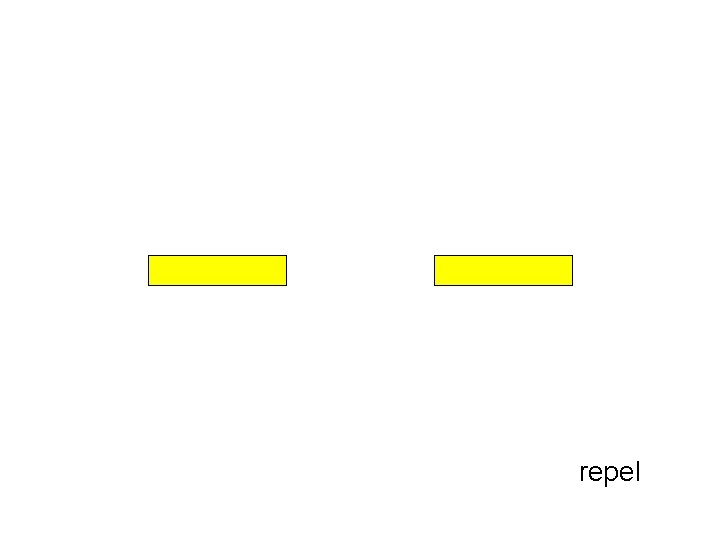repel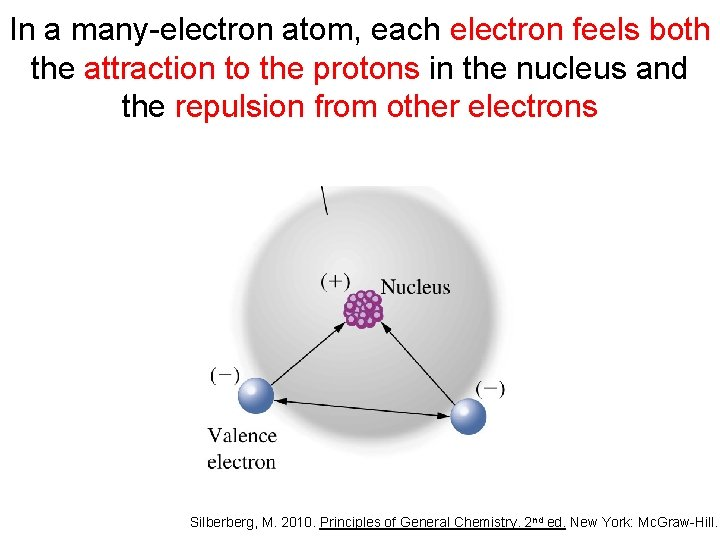In a many-electron atom, each electron feels both the attraction to the protons in the nucleus and the repulsion from other electrons Silberberg, M. 2010. Principles of General Chemistry. 2 nd ed. New York: Mc. Graw-Hill.Effective nuclear charge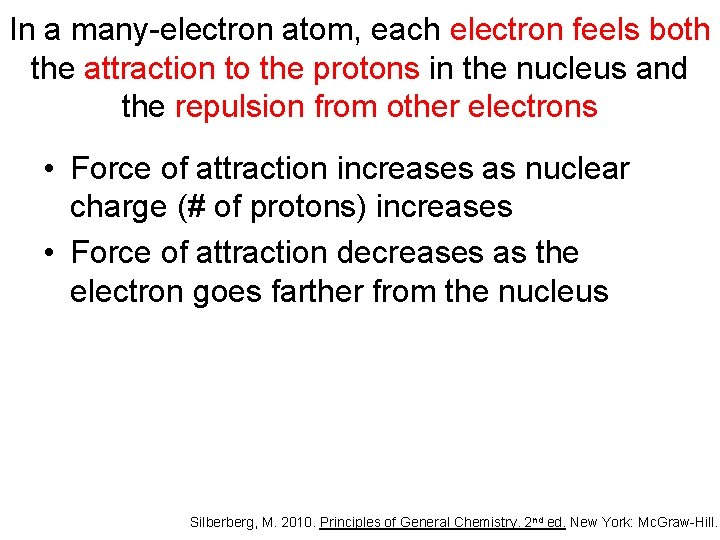In a many-electron atom, each electron feels both the attraction to the protons in the nucleus and the repulsion from other electrons • Force of attraction increases as nuclear charge (# of protons) increases • Force of attraction decreases as the electron goes farther from the nucleus Silberberg, M. 2010. Principles of General Chemistry. 2 nd ed. New York: Mc. Graw-Hill.In effect, the charge felt by an electron is less than the full nuclear charge Silberberg, M. 2010. Principles of General Chemistry. 2 nd ed. New York: Mc. Graw-Hill.In effect, the charge felt by an electron is less than the full nuclear charge • The effective nuclear charge (Zeff) is the nuclear charge that is actually felt by an electron • An electron is shielded from the full charge of the proton – greatly by the inner electrons – only slightly by the other electrons in the same principal quantum number (n) – not at all by outer electrons Silberberg, M. 2010. Principles of General Chemistry. 2 nd ed. New York: Mc. Graw-Hill.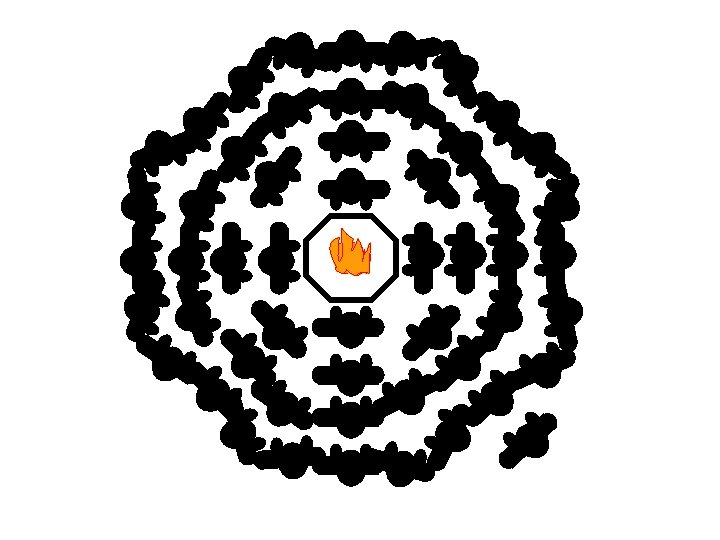The effective nuclear charge and electron configuration are key in understanding the periodic trends • Atomic radius Physical properties • Ionic radius – neutral vs. charged – isoelectronic series • Ionization energy Chemical properties – one atom vs. another – same atom • Electron affinity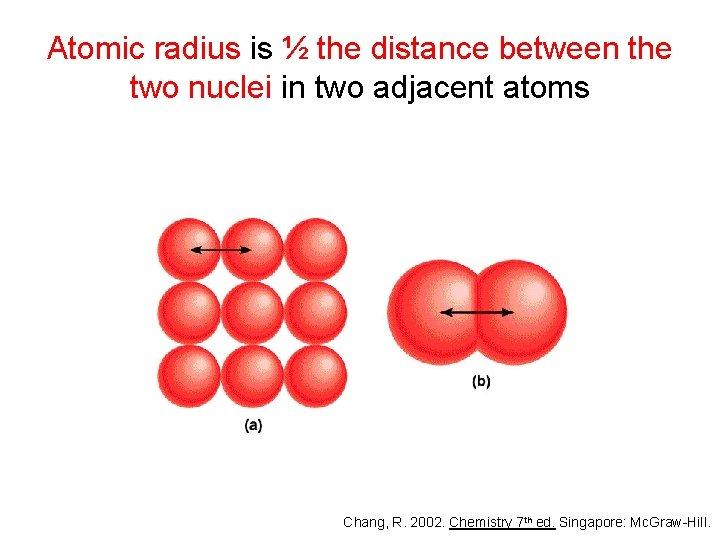Atomic radius is ½ the distance between the two nuclei in two adjacent atoms Chang, R. 2002. Chemistry 7 th ed. Singapore: Mc. Graw-Hill.*Radii in pm Chang, R. 2002. Chemistry 7 th ed. Singapore: Mc. Graw-Hill.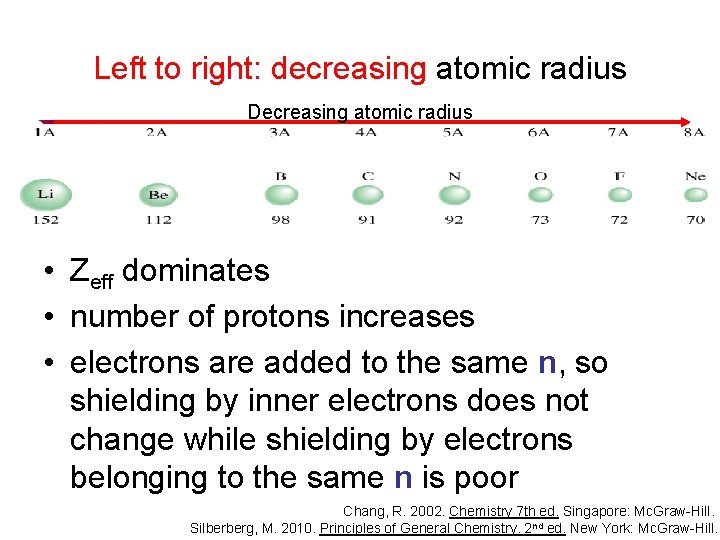Left to right: decreasing atomic radius Decreasing atomic radius • Zeff dominates • number of protons increases • electrons are added to the same n, so shielding by inner electrons does not change while shielding by electrons belonging to the same n is poor Chang, R. 2002. Chemistry 7 th ed. Singapore: Mc. Graw-Hill. Silberberg, M. 2010. Principles of General Chemistry. 2 nd ed. New York: Mc. Graw-Hill.Top to bottom: increasing atomic radius • n dominates • going down the group, each member has one more level of inner electrons that shield the outer electrons very effectively Chang, R. 2002. Chemistry 7 th ed. Singapore: Mc. Graw-Hill. Silberberg, M. 2010. Principles of General Chemistry. 2 nd ed. New York: Mc. Graw-Hill.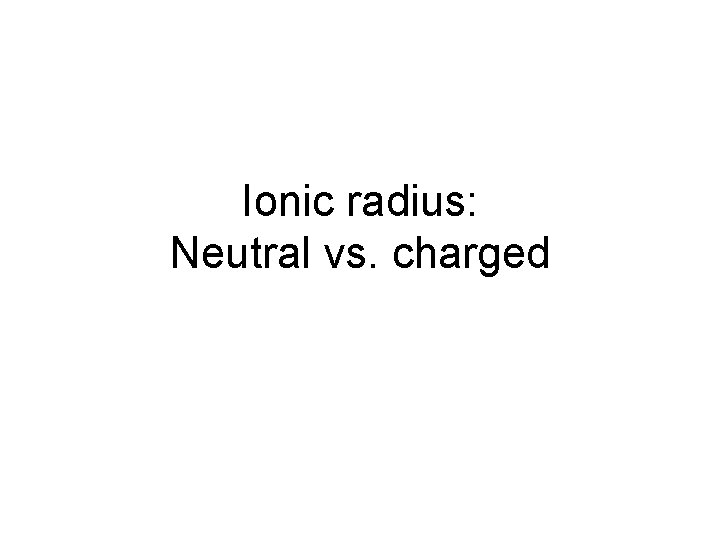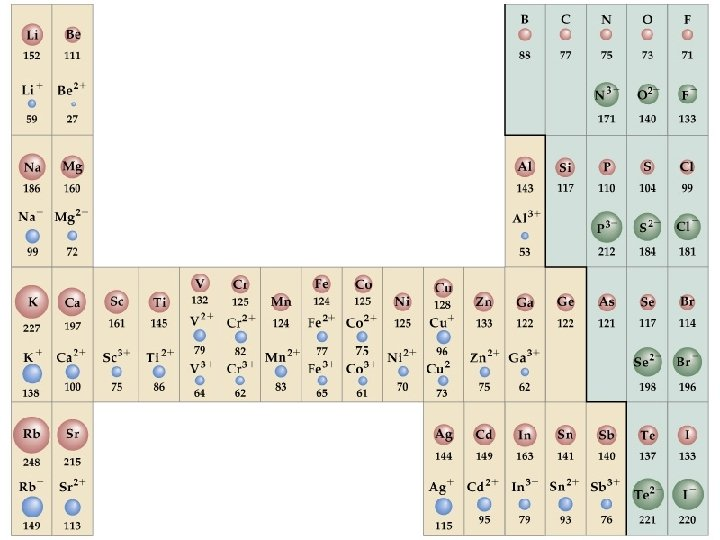If the atom forms a cation, its radius decreases • same number of protons • less electrons • electron-electron repulsion is reduced • the electron cloud becomes smaller Chang, R. 2002. Chemistry 7 th ed. Singapore: Mc. Graw-Hill.If the atom forms an anion, its radius increases • same number of protons • more electrons • electron-electron repulsion is enhanced • the electron cloud becomes bigger Chang, R. 2002. Chemistry 7 th ed. Singapore: Mc. Graw-Hill.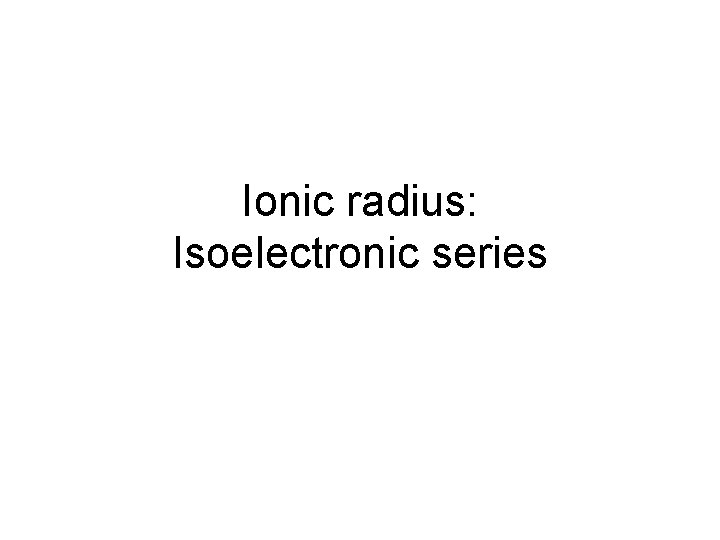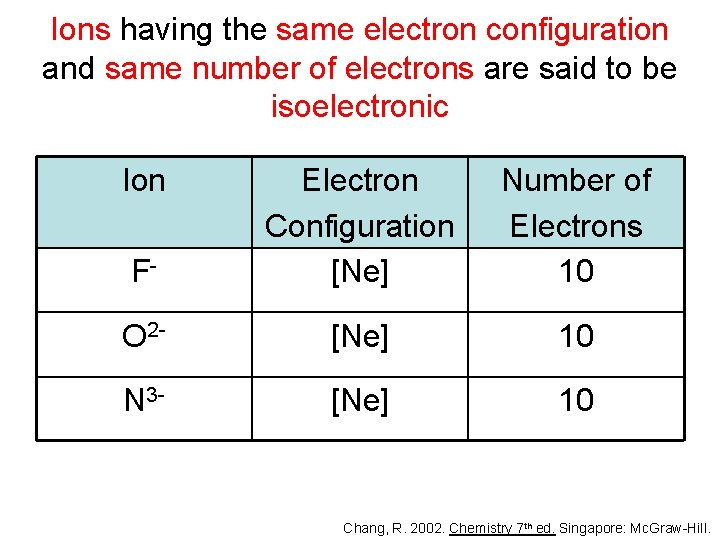Ions having the same electron configuration and same number of electrons are said to be isoelectronic Ion F- Electron Configuration [Ne] Number of Electrons 10 O 2 - [Ne] 10 N 3 - [Ne] 10 Chang, R. 2002. Chemistry 7 th ed. Singapore: Mc. Graw-Hill.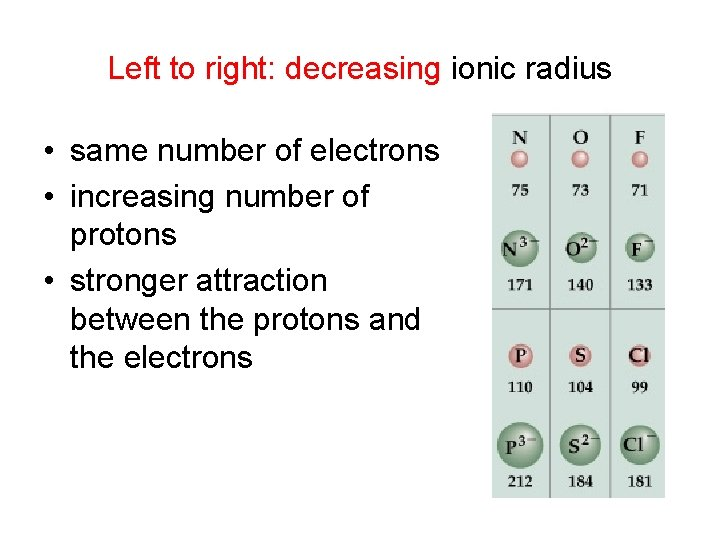Left to right: decreasing ionic radius • same number of electrons • increasing number of protons • stronger attraction between the protons and the electrons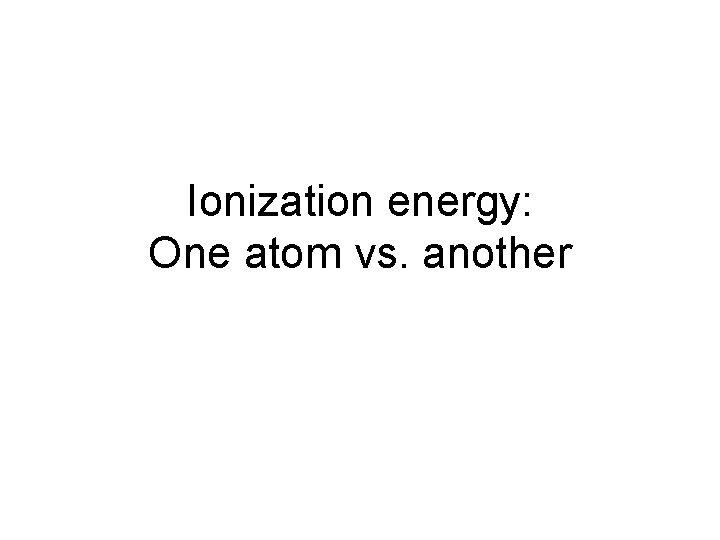Ionization energy: One atom vs. anotherIonization energy (IE) is the minimum energy required to remove an electron from a gaseous atom energy + X(g) X+(g) + e- • The higher the IE, the more difficult it is to remove the electron Chang, R. 2002. Chemistry 7 th ed. Singapore: Mc. Graw-Hill.Brown, , E. Le. May, and B. Bursten. 2000. Chemistry: The Central Science. 8 th ed. Phils: Pearson Education Asia Pte. Ltd.Left to right: increasing ionization energy Increasing ionization energy • • Zeff dominates number of protons increases same n stronger attraction between the protons and the electrons • harder to remove an electron Brown, , E. Le. May, and B. Bursten. 2000. Chemistry: The Central Science. Phils: Pearson Education Asia Pte. Ltd. Chang, R. 2002. Chemistry 7 th ed. Singapore: Mc. Graw-Hill.Decreasing ionization energy Top to bottom: decreasing ionization energy • n dominates • there are more electrons in between the protons and the outer electrons • weaker attraction • easier to remove an electron Brown, , E. Le. May, and B. Bursten. 2000. Chemistry: The Central Science. Phils: Pearson Education Asia Pte. Ltd. Chang, R. 2002. Chemistry 7 th ed. Singapore: Mc. Graw-Hill.Ionization energy: Same atom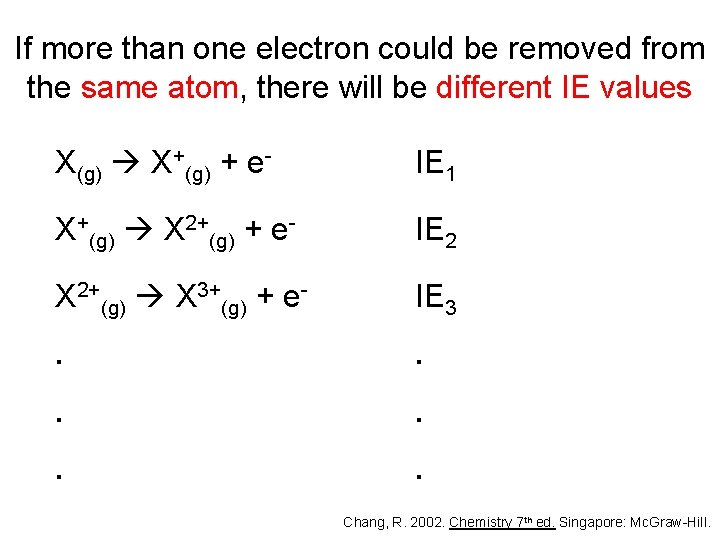If more than one electron could be removed from the same atom, there will be different IE values X(g) X+(g) + e- IE 1 X+(g) X 2+(g) + e- IE 2 X 2+(g) X 3+(g) + e- IE 3 . . . Chang, R. 2002. Chemistry 7 th ed. Singapore: Mc. Graw-Hill.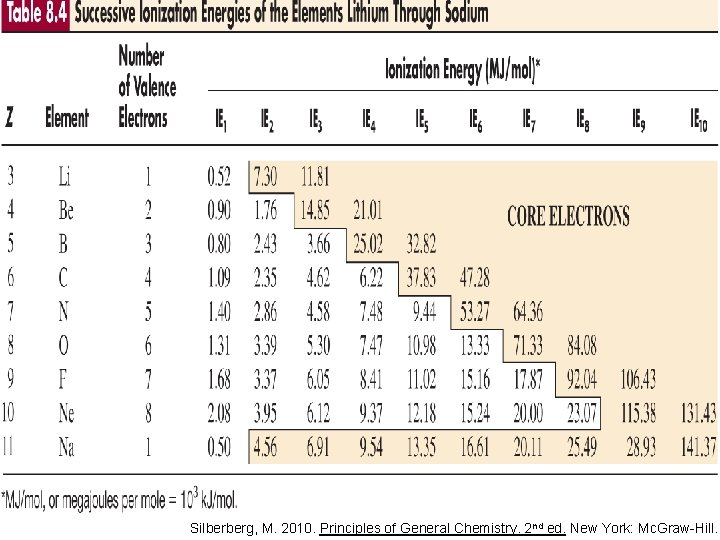Silberberg, M. 2010. Principles of General Chemistry. 2 nd ed. New York: Mc. Graw-Hill.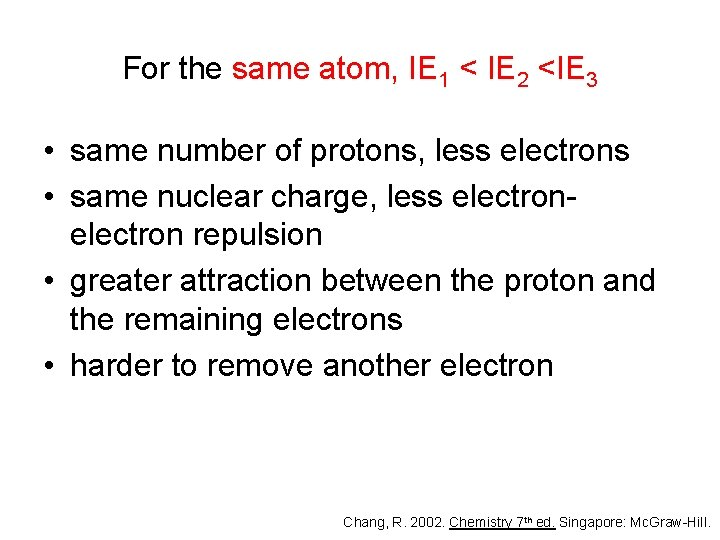For the same atom, IE 1 < IE 2 <IE 3 • same number of protons, less electrons • same nuclear charge, less electron repulsion • greater attraction between the proton and the remaining electrons • harder to remove another electron Chang, R. 2002. Chemistry 7 th ed. Singapore: Mc. Graw-Hill.There is a dramatic increase in IE when an electron is removed from an atom/ion with a noble gas configuration • the noble gas configuration is stable • removing another electron from it will result in instability Chang, R. 2002. Chemistry 7 th ed. Singapore: Mc. Graw-Hill.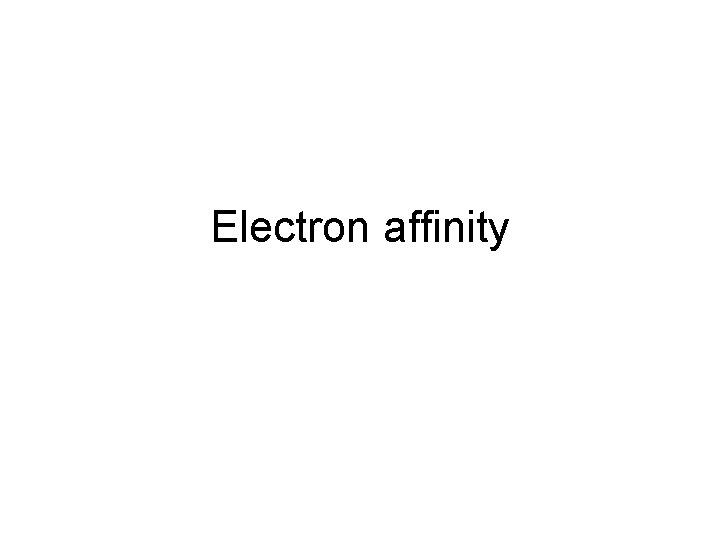Electron affinity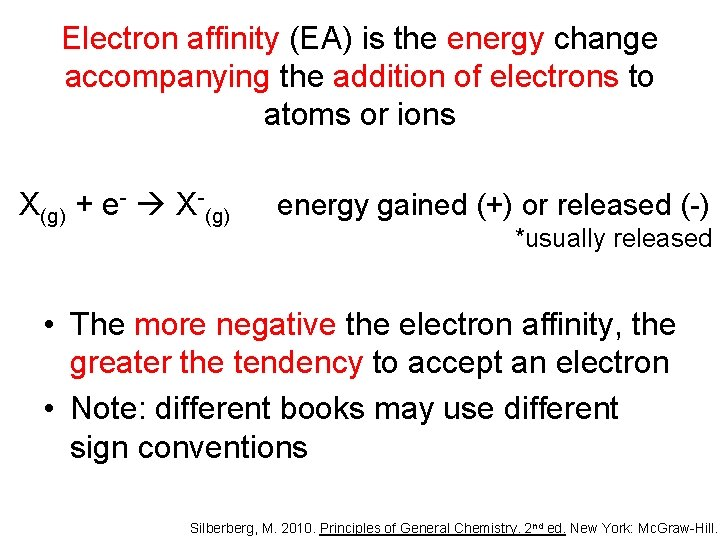Electron affinity (EA) is the energy change accompanying the addition of electrons to atoms or ions X(g) + e- X-(g) energy gained (+) or released (-) *usually released • The more negative the electron affinity, the greater the tendency to accept an electron • Note: different books may use different sign conventions Silberberg, M. 2010. Principles of General Chemistry. 2 nd ed. New York: Mc. Graw-Hill.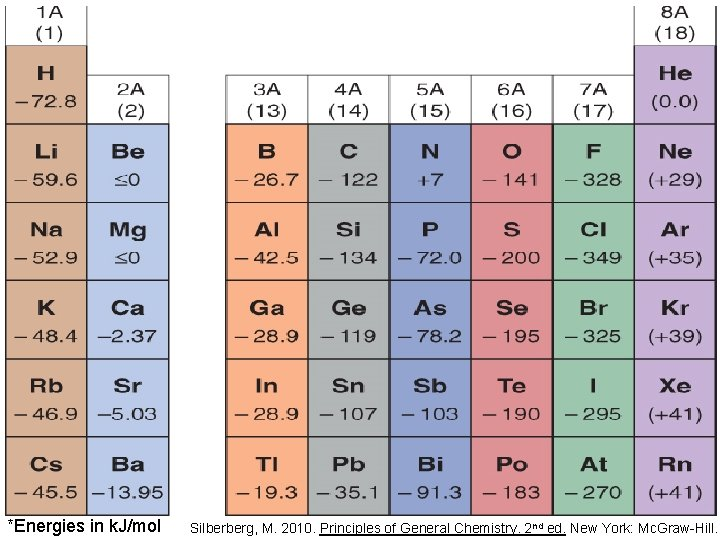*Energies in k. J/mol Silberberg, M. 2010. Principles of General Chemistry. 2 nd ed. New York: Mc. Graw-Hill.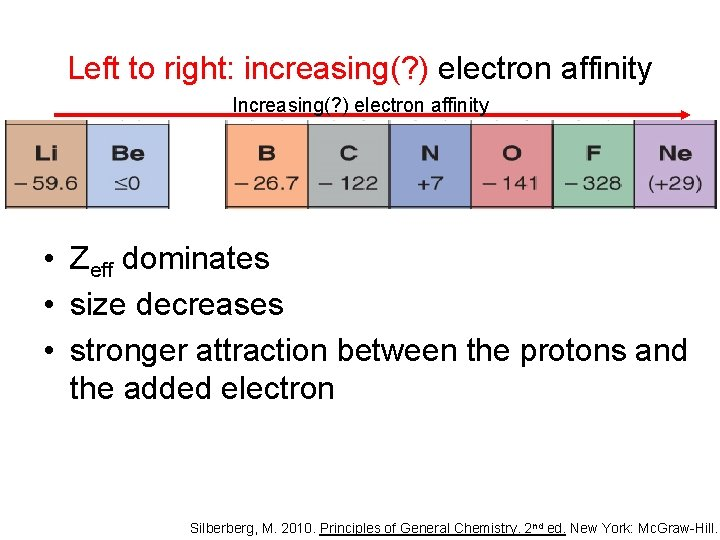Left to right: increasing(? ) electron affinity Increasing(? ) electron affinity • Zeff dominates • size decreases • stronger attraction between the protons and the added electron Silberberg, M. 2010. Principles of General Chemistry. 2 nd ed. New York: Mc. Graw-Hill.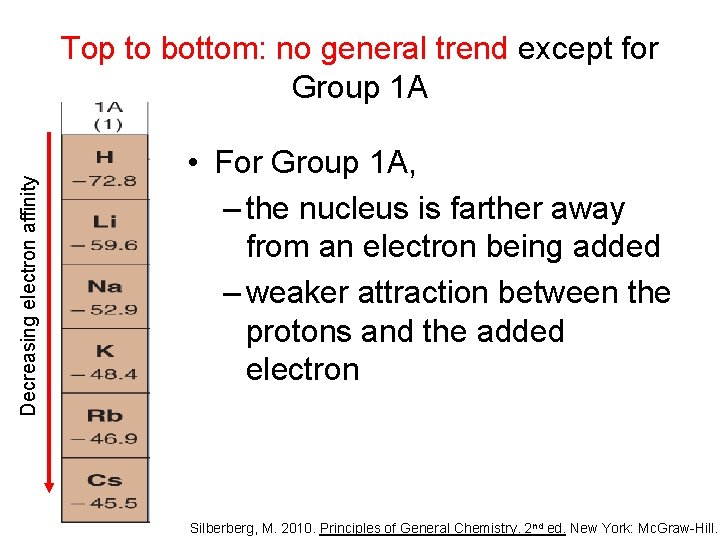Decreasing electron affinity Top to bottom: no general trend except for Group 1 A • For Group 1 A, – the nucleus is farther away from an electron being added – weaker attraction between the protons and the added electron Silberberg, M. 2010. Principles of General Chemistry. 2 nd ed. New York: Mc. Graw-Hill.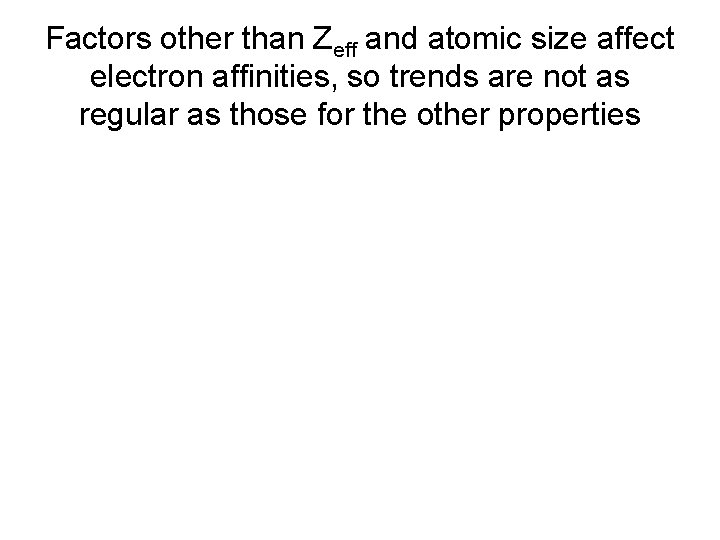Factors other than Zeff and atomic size affect electron affinities, so trends are not as regular as those for the other properties